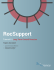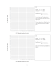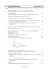A 30.00 milliliter sample of a weak monoprotic acid was... solution of NaOH.

Transcription

A 30.00 milliliter sample of a weak monoprotic acid was... solution of NaOH.
```1988
Tuesday, November 22, 2011
2:04 PM
A 30.00 milliliter sample of a weak monoprotic acid was titrated with a standardized
solution of NaOH.
A pH meter was used to measure the pH after each increment of NaOH was added, and
the curve above was constructed.
(a) Explain how this curve could be used to determine the molarity of the acid.
(b) Explain how this curve could be used to determine the dissociation constant Ka of
the weak monoprotic acid.
(c) If you were to repeat the titration using a indicator in the acid to signal the endpoint,
which of the following indicators should you select? Give the reason for your choice.
Methyl red Ka = 1 × 10–5
Cresol red Ka = 1 × 10–8
Alizarin yellow Ka = 1 × 10–11
(d) Sketch the titration curve that would result if the weak monoprotic acid were
replaced by a strong monoprotic acid, such as HCl of the same molarity. Identify
differences between this titration curve and the curve shown above.
Free Response Page 1
```

Parabolic CurveJuvenile Idiopathic Scoliosis Reference Guide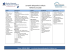3 sample exam paper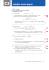C1 prep set mock week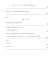Economics Assignment - dav international school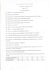Economics 2301-03 SAMPLE QUESTIONS FOR MIDTERM EXAM #2 Fall, 2011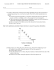Paper G QP â C2 Solomon - Mathematics Christopher Page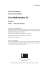Math 1172 Sample Test #1 + 1 and the line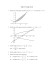Ballasted R40 Curve Baseplates - L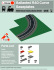lesto - Dux Lighting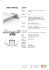03.26.15 HW27 Writing Sinusoidal Functions to Model Simple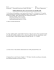STANDARD NORMAL DISTRIBUTIONwhere the girls are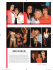C2 Worksheet D - WordPress.com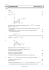Document 6537674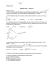Economics 151 Development Economics Sample Exam Questions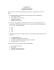Worksheet buffers 2014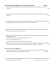CBSE Maths Sample Paper for Class 12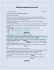MDM4U - Normal Distribution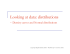Long Term Ground Reaction